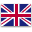UFO Battery is a professional supplier of lithium-ion batteries and power solutions.English
Home > RESOURCES > Knowledge Base > How long will a 100ah battery run a fridge?
Bll Categories
More
Latest Blog
More
Blog Tag
More

# How long will a 100ah battery run a fridge?

by：hqt

In another article about calculating the time a 12 volt battery run a refrigerator, we know that there are two kinds of refrigerators which is residential refrigerator rated at 110-220V or the RV fridge rated at 12V for camping. What about the situation when the battery is 100ah?

Suppose both the residential and RV refrigerators in the following examples are mid-sized energy star certified refrigerators and the load factor is 80%. The residential refrigerator has an initial spike of 720 watts and consumes 240 watts regularly, and the RV fridge is rated at 250W for running and 750 watts for the spike starting. As a result, the inverter capacity should be at least 1500 watts for the two refrigerators, three times the refrigerator’s starting power.

For the lithium ion battery case, 80% DOD is applied.

• 110-220V residential refrigerator

The required battery rating is supposed to be 1500/100/80%=18.75 volt at least. Considering the battery voltage can be 24V or 48V for common cases, the lithium ion battery can be 48V for longer time, in which case the battery power (Wh)= battery capacity (AH) X battery voltage (V) X number of batteries = 100 X 48 X 1 = 4800 Watts. As a result, the backup time is equal to battery power (Wh) / connected load (W) = 4800/240=20 hours theoretically.• 12V RV fridge

If the refrigerator is the 12V RV one, then the 100ah battery is rated at 1500/100/80%=18.75 volt, and the backup time is battery power (Wh) / connected load (W) = 4800/250=19 hours theoretically.

In case the battery is the lead-acid one, 50% DOD is applied.

• 110-220V residential refrigerator

The required battery rating is supposed to be 1500/100/50%=30 volts. As a result, battery power is 100 X 30 X 1 = 3000 Watts, and thus the backup time is 3000/240=12.5 hours theoretically.

• 12V RV fridgeThe required battery voltage is at least 1500/100/50%=30 volts, and thus the required battery power is the same as the example of above residential refrigerator. The backup time is battery power/fridge power=3000/250= 12 hours.

Chat Online 编辑模式下无法使用
Chat Online inputting...